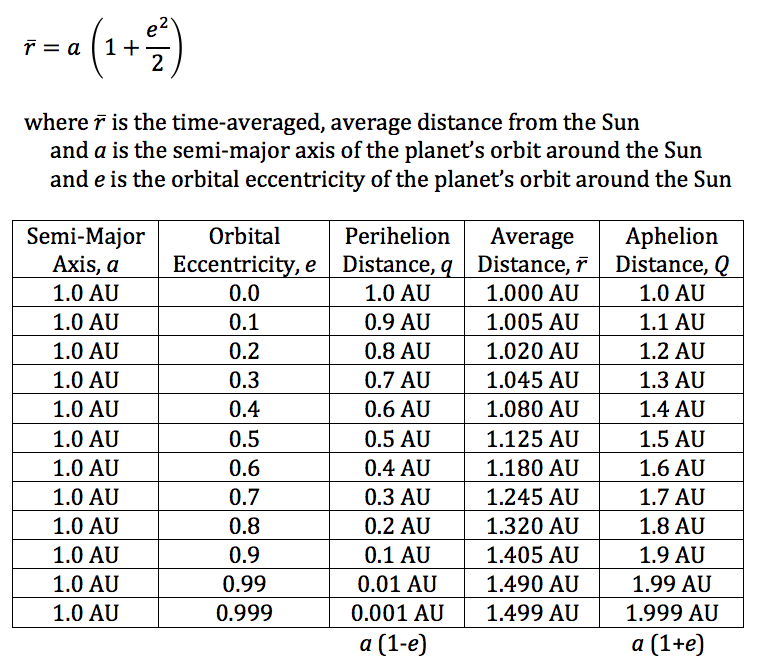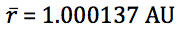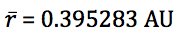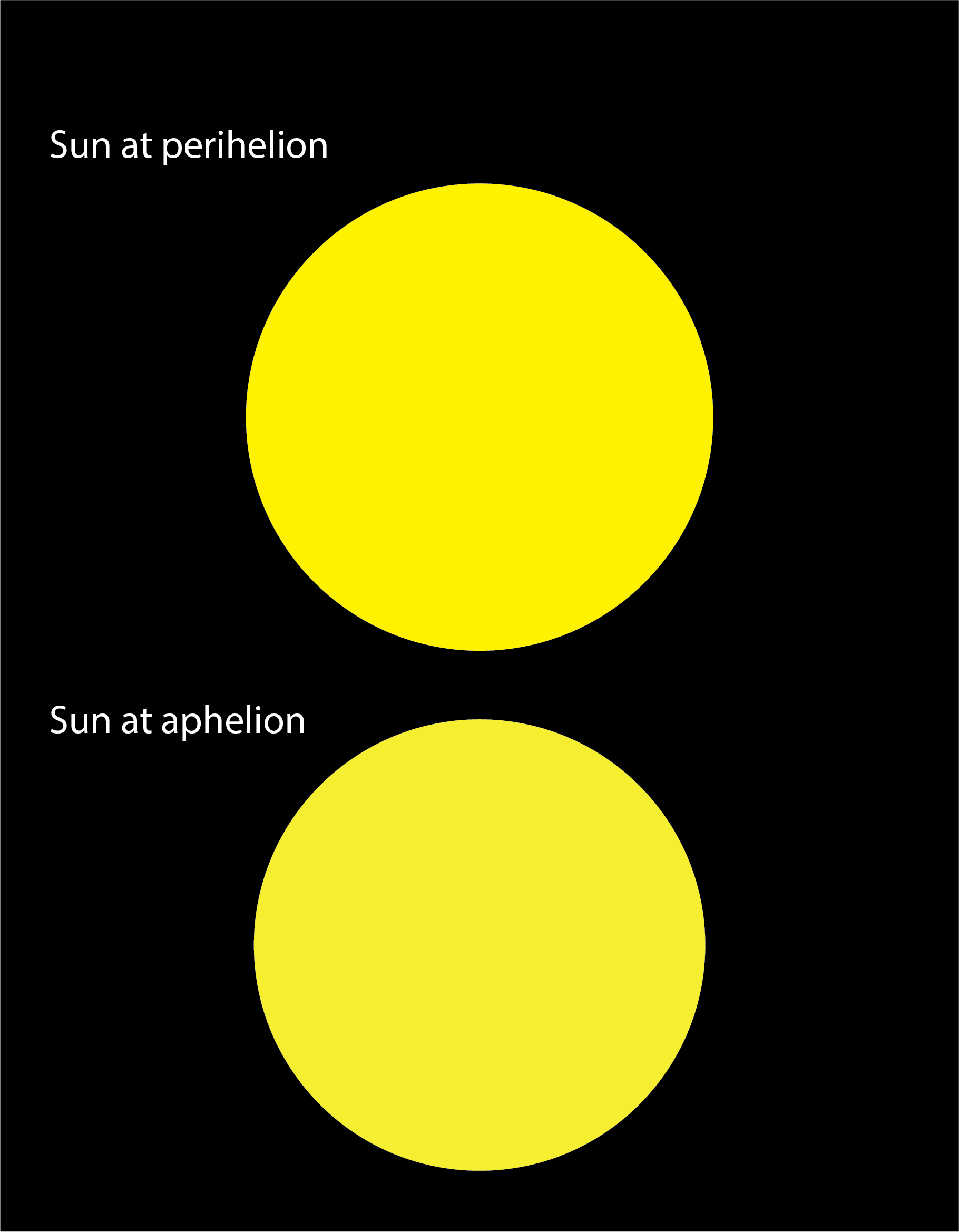## Mercury, Our Nearest Planetary Neighbor

If you’re an astronomy teacher that likes to put a trick question on an open book quiz or test once in a while to encourage your students to think more deeply, here’s a good one for you:

On average, what planet is closest to the Earth?

A. Mars

B. Venus

C. Mercury

The correct answer is C. Mercury.

Huh? Venus comes closest to the Earth, doesn’t it? Yes, but there is a big difference between minimum distance and average distance. Let’s do some quick calculations to help us understand minimum distance first, and then we’ll discuss the more involved determination of average distance.

Here’s some easily-found data on the terrestrial planets:

 Planet a e q Q Mercury 0.387 0.206 Venus 0.723 0.007 Earth 1.000 0.017 Mars 1.524 0.093

I’ve intentionally left the last two columns of the table empty. We’ll come back to those in a moment. a is the semi-major axis of each planet’s orbit around the Sun, in astronomical units (AU). It is often taken that this is the planet’s average distance from the Sun, but that is strictly true only for a circular orbit.1 e is the orbital eccentricity, which is a unitless number. The closer the value is to 0.0, the more circular the orbit. The closer the value is to 1.0, the more elliptical the orbit, with 1.0 being a parabola.

The two empty columns are for q the perihelion distance, and Q the aphelion distance. Perihelion occurs when the planet is closest to the Sun. Aphelion occurs when the planet is farthest from the Sun. How do we calculate the perihelion and aphelion distance? It’s easy.

Perihelion: q = a (1 – e)

Aphelion: q = a (1 + e)

Now, let’s fill in the rest of our table.

 Planet a (AU) e q (AU) Q (AU) Mercury 0.387 0.206 0.307 0.467 Venus 0.723 0.007 0.718 0.728 Earth 1.000 0.017 0.983 1.017 Mars 1.524 0.093 1.382 1.666

Ignoring, for a moment, each planet’s orbital eccentricity, we can calculate the “average” closest approach distance between any two planets by simply taking the difference in their semi-major axes. For Venus, it is 1.000 – 0.723 = 0.277 AU, and for Mars, it is 1.524 – 1.000 = 0.524 AU. We see that Venus comes closest to the Earth.

But, sometimes, Venus and Mars come even closer to the Earth than 0.277 AU and 0.524 AU, respectively. The minimum minimum distance between Venus and the Earth in conjunction should occur when Venus is at aphelion at the same time as Earth is at perihelion: 0.983 – 0.728 = 0.255 AU. The minimum minimum distance between Earth and Mars at opposition should occur when Mars is at perihelion and Earth is at aphelion: 1.382 – 1.017 = 0.365 AU. Mars does not ever come as close to the Earth as Venus does at every close approach.

The above assumes that all the terrestrial planets orbit in the same plane, which they do not. Mercury has an orbital inclination relative to the ecliptic of 7.004˚, Venus 3.395˚, Earth 0.000˚ (by definition), and Mars 1.848˚. Calculating the distances in 3D will change the values a little, but not by much.

Now let’s switch gears and find the average distance over time between Earth and the other terrestrial planets—a very different question. But we want to pick a time period to average over that is sufficiently long enough that each planet spends as much time on the opposite side of the Sun from us as it does on our side of the Sun. The time interval between successive conjunctions (in the case of Mercury and Venus) or oppositions (Mars) is called the synodic period and is calculated as follows:

P1 = 87.9691d = orbital period of Mercury

P2 = 224.701d = orbital period of Venus

P3 = 365.256d = orbital period of Earth

P4 = 686.971d = orbital period of Mars

S1 = (P1-1 – P3-1)-1 = synodic period of Mercury = 115.877d

S2 = (P2-1 – P3-1)-1 = synodic period of Venus = 583.924d

S4 = (P3-1 – P4-1)-1 = synodic period of Mars = 779.946d

I wrote a quick little SAS program to numerically determine that an interval of 9,387 days (25.7 years) would be a good choice, because

9387 / 115.877 = 81.0083, for Mercury

9387 / 583.924 = 16.0757, for Venus

9387 / 779.946 = 12.0354, for Mars

The U.S Naval Observatory provides a free computer program called the Multiyear Interactive Computer Almanac (MICA), so I was able to quickly generate a file for each planet, Mercury, Venus, and Mars, giving the Earth-to-planet distance for 9,387 days beginning 0h UT 1 May 2019 through 0h UT 10 Jan 2045. Here are the results:

 Planet Mean (AU) Median (AU) Min (AU) Max (AU) Mercury 1.039022 1.073148 0.549144 1.451501 Venus 1.138383 1.238453 0.265260 1.735280 Mars 1.711176 1.842260 0.380420 2.675330

As you can see, averaged over time, Mercury is the nearest planet to the Earth!

For a more mathematical treatment, see the article in the 12 Mar 2019 issue of Physics Today.

1 See my article Average Orbital Distance for details.

## Like Sun, Like Moon

The Earth orbits the Sun once every 365.256363 (mean solar) days relative to the distant stars.  The Earth’s orbital speed ranges from 18.2 miles per second at aphelion, around July 4th, to 18.8 miles per second at perihelion, around January 3rd.  In units we’re perhaps more familiar with, that’s 65,518 mph at aphelion and 67,741 mph at perihelion. That’s a difference of 2,223 miles per hour!

As we are on a spinning globe, the direction towards which the Earth is orbiting is different at different times of the day.  When the Sun crosses the celestial meridian, due south, at its highest point in the sky around noon (1:00 p.m. daylight time), the Earth is orbiting towards your right (west) as you are facing south. Since the Earth is orbiting towards the west, the Sun appears to move towards the east, relative to the background stars—if we could see them during the day.  Since there are 360° in a circle and the Earth orbits the Sun in 365.256363 days (therefore the Sun appears to go around the Earth once every 365.256363 days relative to the background stars), the Sun’s average angular velocity eastward relative to the background stars is 360°/365.256363 days = 0.9856° per day.

The constellations through which the Sun moves are called the zodiacal constellations, and historically the zodiac contained 12 constellations, the same number as the number of months in a year.  But Belgian astronomer Eugène Delporte (1882-1955) drew up the 88 constellation boundaries we use today, approved by the IAU in 1930, so now the Sun spends a few days each year in the non-zodiacal constellation Ophiuchus, the Serpent Bearer. Furthermore, because the Earth’s axis is precessing, the calendar dates during which the Sun is in a particular zodiacal constellation is gradually getting later.

Astrologically, each zodiacal constellation has a width of 30° (360° / 12 constellations = 30° per constellation).  But, of course, the constellations are different sizes and shapes, so astronomically the number of days the Sun spends in each constellation varies. Here is the situation at present.

Constellation

Description

Sun Travel Dates

Capricornus

Sea Goat

Jan 19 through Feb 16

Aquarius

Water Bearer

Feb 16 through Mar 12

Pisces

The Fish

Mar 12 through Apr 18

Aries

The Ram

Apr 18 through May 14

Taurus

The Bull

May 14 through Jun 21

Gemini

The Twins

Jun 21 through Jul 20

Cancer

The Crab

Jul 20 through Aug 10

Leo

The Lion

Aug 10 through Sep 16

Virgo

The Virgin

Sep 16 through Oct 31

Libra

The Scales

Oct 31 through Nov 23

Scorpius

The Scorpion

Nov 23 through Nov 29

Ophiuchus

Serpent Bearer

Nov 29 through Dec 18

Sagittarius

The Archer

Dec 18 through Jan 19

The apparent path the Sun takes across the sky relative to the background stars through these 13 constellations is called the ecliptic.  A little contemplation, aided perhaps by a drawing, will convince you that the ecliptic is also the plane of the Earth’s orbit around the Sun.  The Moon never strays very far from the ecliptic in our sky, since its orbital plane around the Earth is inclined at a modest angle of 5.16° relative to the Earth’s orbital plane around the Sun.  But, relative to the Earth’s equatorial plane, the inclination of the Moon’s orbit varies between 18.28° and 28.60° over 18.6 years as the line of intersection between the Moon’s orbital plane and the ecliptic plane precesses westward along the ecliptic due to the gravitational tug of war the Earth and the Sun exert on the Moon as it moves through space.  This steep inclination to the equatorial plane is very unusual for such a large moon.  In fact, all four satellites in our solar system that are larger than our Moon (Ganymede, Titan, Callisto, and Io) and the one that is slightly smaller (Europa) all orbit in a plane that is inclined less than 1/2° from the equatorial plane of their host planet (Jupiter and Saturn).

Since the Moon is never farther than 5.16° from the ecliptic, its apparent motion through our sky as it orbits the Earth mimics that of the Sun, only the Moon’s angular speed is over 13 times faster, completing its circuit of the sky every 27.321662 days, relative to the distant stars.  Thus the Moon moves a little over 13° eastward every day, or about 1/2° per hour.  Since the angular diameter of the Moon is also about 1/2°, we can easily remember that the Moon moves its own diameter eastward relative to the stars every hour.  Of course, superimposed on this motion is the 27-times-faster-yet motion of the Moon and stars westward as the Earth rotates towards the east.

Now, take a look at the following table and see how the Moon’s motion mimics that of the Sun throughout the month, and throughout the year.

——— Moon’s Phase and Path ———

Date

Sun’s Path

New

FQ

Full

LQ

Mar 20

EQ

EQ

High

EQ

Low

Jun 21

High

High

EQ

Low

EQ

Sep 22

EQ

EQ

Low

EQ

High

Dec 21

Low

Low

EQ

High

EQ

New = New Moon

near the Sun

FQ = First Quarter

90° east of the Sun

Full = Full Moon

180°, opposite the Sun

LQ = Last Quarter

90° west of the Sun

EQ

= crosses the celestial equator heading north

High

= rides high (north) across the sky

EQ

= crosses the celestial equator heading south

Low

= rides low (south) across the sky

So, if you aren’t already doing so, take note of how the Moon moves across the sky at different phases and times of the year.  For example, notice how the full moon (nearest the summer solstice) on June 27/28 rides low in the south across the sky.  You’ll note the entry for the “Jun 21” row and “Full” column is “Low”.  And, the Sun entry for that date is “High”.  See, it works!

## Average Orbital Distance

If a planet is orbiting the Sun with a semi-major axis, a, and orbital eccentricity, e, it is often stated that the average distance of the planet from the Sun is simply a.  This is only true for circular orbits (e = 0) where the planet maintains a constant distance from the Sun, and that distance is a.

Let’s imagine a hypothetical planet much like the Earth that has a perfectly circular orbit around the Sun with a = 1.0 AU and e = 0.  It is easy to see in this case that at all times, the planet will be exactly 1.0 AU from the Sun.

If, however, the planet orbits the Sun in an elliptical orbit at a = 1 AU and e > 0, we find that the planet orbits more slowly when it is farther from Sun than when it is nearer the Sun.  So, you’d expect to see the time-averaged average distance to be greater than 1.0 AU.  This is indeed the case.The Earth’s current osculating orbital elements give us:

a = 0.999998 and e = 0.016694

Earth’s average distance from the Sun is thus:Mercury, the innermost planet, has the most eccentric orbit of all the major planets:

a = 0.387098 and e = 0.205638

Mercury’s average distance from the Sun is thus:## Changing Solar Distance

Between January 2 and 5 each year, the Earth reaches orbital perihelion, its closest distance to the Sun (0.983 AU).  Between July 3 and 6 each year, the Earth reaches orbital aphelion, its farthest distance from the Sun (1.017 AU).  These dates of perihelion and aphelion slowly shift across the calendar (always a half year apart) with a period between 22,000 and 26,000 years.

These distances can be easily derived knowing the semi-major axis (a) and orbital eccentricity (e) of the Earth’s orbit around the Sun, which are 1.000 and 0.017, respectively.

perihelion
q = a (1-e) = 1.000 (1-0.017) = 0.983 AU

aphelion
Q = a (1+e) = 1.000 (1+0.017) = 1.017 AU

So, the Earth is 0.034 AU closer to the Sun in early January than it is in early July.  This is about 5 million km or 3.1 million miles.

How great a distance is this, really?  The Moon in its orbit around the Earth is closer to the Sun around New Moon than it is around Full Moon.  Currently, this difference in distance ranges between 130,592 miles (April 2018) and 923,177 miles (October 2018).  Using the latter value, we see that the Moon’s maximum monthly range in distance from the Sun is 30% of the Earth’s range in distance from the Sun between perihelion and aphelion.

How about in terms of the diameter of the Sun?  The Sun’s diameter is 864,526 miles.  The Earth is just 3.6 Sun diameters closer to the Sun at perihelion than it is at aphelion.  Not much!  On average, the Earth is about 108 solar diameters distant from the Sun.

How about in terms of angular size?  When the Earth is at perihelion, the Sun exhibits an angular size of 29.7 arcminutes.  At aphelion, that angle is 28.7 arcminutes.Can you see the difference?